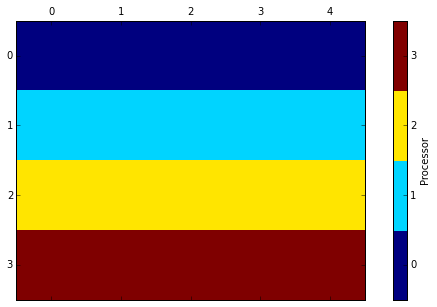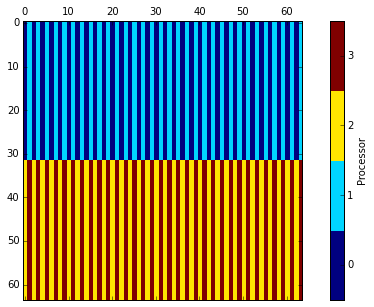DistArray: Distributed Arrays for Python¶

Robert Grant, Enthought

11 June 2014

github.com/enthought/distarray

Start a cluster¶

These examples require an IPython.parallel cluster to be running. Outside the notebook, run

dacluster start -n4
In :
# some utility imports
from pprint import pprint
from matplotlib import pyplot as plt

# main imports
import numpy
import distarray

numpy.set_printoptions(precision=2)  # display formatting

NumPy Arrays¶

In :
nparr = numpy.random.random((4, 5))
nparr
Out:
array([[ 0.67,  0.27,  0.91,  0.05,  0.78],
[ 0.38,  0.07,  0.15,  0.7 ,  0.64],
[ 0.82,  0.18,  0.58,  0.14,  0.97],
[ 0.76,  0.88,  0.5 ,  0.34,  0.89]])
In :
# NumPy array attributes
print "type:", type(nparr)
print "dtype:", nparr.dtype
print "ndim:", nparr.ndim
print "shape:", nparr.shape
print "itemsize:", nparr.itemsize
print "nbytes:", nparr.nbytes
type: <type 'numpy.ndarray'>
dtype: float64
ndim: 2
shape: (4, 5)
itemsize: 8
nbytes: 160

DistArrays¶

In :
from distarray.globalapi import Context
context = Context()
darr = context.fromarray(nparr)
darr
Out:
<DistArray(shape=(4, 5), targets=[0, 1, 2, 3])>
In :
# parts of the array are stored on each engine
for i, a in enumerate(darr.get_localarrays()):
print i, a
0 [[ 0.67  0.27  0.91  0.05  0.78]]
1 [[ 0.38  0.07  0.15  0.7   0.64]]
2 [[ 0.82  0.18  0.58  0.14  0.97]]
3 [[ 0.76  0.88  0.5   0.34  0.89]]
In :
# DistArray attributes
print "type:", type(darr)
print "dtype:", darr.dtype
print "ndim:", darr.ndim
print "shape:", darr.shape
print "itemsize:", darr.itemsize
print "nbytes:", darr.nbytes
type: <class 'distarray.globalapi.distarray.DistArray'>
dtype: float64
ndim: 2
shape: (4, 5)
itemsize: 8
nbytes: 160
In :
# with some extra...
print "targets:", darr.targets
print "context:", darr.context
print "distribution:", darr.distribution
targets: [0, 1, 2, 3]
context: <distarray.globalapi.context.Context object at 0x1065a33d0>

Universal Functions (ufuncs)¶

In :
## NumPy ##
numpy.sin(nparr)
Out:
array([[ 0.62,  0.27,  0.79,  0.05,  0.71],
[ 0.37,  0.07,  0.15,  0.65,  0.59],
[ 0.73,  0.18,  0.55,  0.14,  0.82],
[ 0.69,  0.77,  0.48,  0.34,  0.77]])
In :
## DistArray ##
import distarray.globalapi as da
da.sin(darr)
Out:
<DistArray(shape=(4, 5), targets=[0, 1, 2, 3])>
In :
da.sin(darr).toarray()
Out:
array([[ 0.62,  0.27,  0.79,  0.05,  0.71],
[ 0.37,  0.07,  0.15,  0.65,  0.59],
[ 0.73,  0.18,  0.55,  0.14,  0.82],
[ 0.69,  0.77,  0.48,  0.34,  0.77]])
In :
## NumPy ##
nparr + nparr
Out:
array([[ 1.33,  0.55,  1.82,  0.1 ,  1.57],
[ 0.77,  0.14,  0.3 ,  1.41,  1.27],
[ 1.64,  0.36,  1.16,  0.28,  1.94],
[ 1.52,  1.76,  1.01,  0.69,  1.77]])
In :
## DistArray ##
darr + darr
Out:
<DistArray(shape=(4, 5), targets=[0, 1, 2, 3])>
In :
(darr + darr).toarray()
Out:
array([[ 1.33,  0.55,  1.82,  0.1 ,  1.57],
[ 0.77,  0.14,  0.3 ,  1.41,  1.27],
[ 1.64,  0.36,  1.16,  0.28,  1.94],
[ 1.52,  1.76,  1.01,  0.69,  1.77]])

Distributions¶

In :
# Distributions control which processes own which (global) indices
distribution = darr.distribution
In :
# this is a 2D distribution
pprint(distribution.maps)
In :
# setup
from distarray.plotting import plot_array_distribution
process_coords = [(0, 0), (1, 0), (2, 0), (3, 0)]
In :
plot_array_distribution(darr, process_coords, cell_label=False, legend=True)
Out:
<DistArray(shape=(4, 5), targets=[0, 1, 2, 3])>In :
distribution.maps.bounds
Out:
[(0, 1), (1, 2), (2, 3), (3, 4)]
In :
# the above is the default, you can make more complex distributions
from distarray.globalapi import Distribution
distribution = Distribution.from_shape(context, (64, 64), dist=('b', 'c'))
a = context.zeros(distribution, dtype='int32')
plot_array_distribution(a, process_coords, cell_label=False, legend=True)
Out:
<DistArray(shape=(64, 64), targets=[0, 1, 2, 3])>Contexts¶

In :
# Context objects manage the setup and communication of the worker processes
# for DistArray objects.
print "targets:", context.targets
print "comm:", context.comm
targets: [0, 1, 2, 3]
comm: __distarray__e60fe9953c015b300

IO Support (v0.2)¶

In :
# load .npy files in parallel
numpy.save("/tmp/outfile.npy", nparr)
distribution = Distribution.from_shape(context, nparr.shape)
new_darr
Out:
<DistArray(shape=(4, 5), targets=[0, 1, 2, 3])>
In :
# save DistArrays to .hdf5 files in parallel
context.save_hdf5("/tmp/outfile.hdf5", darr, mode='w')
In :
# load DistArrays from .hdf5 files in parallel (using h5py)
Out:
<DistArray(shape=(4, 5), targets=[0, 1, 2, 3])>
In :
# save to .dnpy (a built-in flat-file format based on .npy)
context.save_dnpy("/tmp/outfile", darr)
In :
Out:
<DistArray(shape=(4, 5), targets=[0, 1, 2, 3])>

Reductions (v0.3)¶

In :
## NumPy ##
print "sum:", nparr.sum()
print "sum over an axis:", nparr.sum(axis=1)
sum: 10.6867002882
sum over an axis: [ 2.68  1.94  2.69  3.37]
In :
## DistArray ##
print "sum:", darr.sum(), darr.sum().toarray()
print "sum over an axis:", darr.sum(axis=1), darr.sum(axis=1).toarray()
sum: <DistArray(shape=[], targets=)> 10.6867002882
sum over an axis: <DistArray(shape=, targets=[0, 1, 2, 3])> [ 2.68  1.94  2.69  3.37]

Context.apply (v0.3)¶

Global view, local control

In :
def get_local_random():
import numpy
return numpy.random.randint(10)

context.apply(get_local_random)
Out:
[2, 4, 6, 5]
In :
def get_local_var(darr):
return darr.ndarray.var()

context.apply(get_local_var, args=(darr.key,))
Out:
[0.1043967698165971,
0.064006352164566974,
0.11124749755337127,
0.046561405334938577]

Distributed Slicing (v0.4)¶

(coming soon)

In :
# as a reminder
darr.toarray()
Out:
array([[ 0.67,  0.27,  0.91,  0.05,  0.78],
[ 0.38,  0.07,  0.15,  0.7 ,  0.64],
[ 0.82,  0.18,  0.58,  0.14,  0.97],
[ 0.76,  0.88,  0.5 ,  0.34,  0.89]])
In :
darr.get_localshapes()
Out:
[(1, 5), (1, 5), (1, 5), (1, 5)]
In :
# take a column slice
darr_view = darr[:, 3]
print darr_view
print darr_view.toarray()
<DistArray(shape=(4,), targets=[0, 1, 2, 3])>
[ 0.05  0.7   0.14  0.34]
In :
# changes in the view change the original
darr_view = -0.99
print "view:"
print darr_view.toarray()

print "original:"
print darr.toarray()
view:
[ 0.05  0.7   0.14 -0.99]
original:
[[ 0.67  0.27  0.91  0.05  0.78]
[ 0.38  0.07  0.15  0.7   0.64]
[ 0.82  0.18  0.58  0.14  0.97]
[ 0.76  0.88  0.5  -0.99  0.89]]
In :
# a more complex slice
print darr[:, 2::2]
print darr[:-1, 2::2].toarray()
<DistArray(shape=(4, 2), targets=[0, 1, 2, 3])>
[[ 0.91  0.78]
[ 0.15  0.64]
[ 0.58  0.97]]

Distributed Array Protocol (v0.2)¶

(exporting and importing distributed arrays)

In :
def return_protocol_structure(darr):
return darr.__distarray__()

context.apply(return_protocol_structure, args=(darr.key,))
Out:
[{'__version__': '0.10.0',
'buffer': array([[ 0.67,  0.27,  0.91,  0.05,  0.78]]),
'dim_data': ({'dist_type': 'b',
'proc_grid_rank': 0,
'proc_grid_size': 4,
'size': 4,
'start': 0,
'stop': 1},
{'dist_type': 'b',
'proc_grid_rank': 0,
'proc_grid_size': 1,
'size': 5,
'start': 0,
'stop': 5})},
{'__version__': '0.10.0',
'buffer': array([[ 0.38,  0.07,  0.15,  0.7 ,  0.64]]),
'dim_data': ({'dist_type': 'b',
'proc_grid_rank': 1,
'proc_grid_size': 4,
'size': 4,
'start': 1,
'stop': 2},
{'dist_type': 'b',
'proc_grid_rank': 0,
'proc_grid_size': 1,
'size': 5,
'start': 0,
'stop': 5})},
{'__version__': '0.10.0',
'buffer': array([[ 0.82,  0.18,  0.58,  0.14,  0.97]]),
'dim_data': ({'dist_type': 'b',
'proc_grid_rank': 2,
'proc_grid_size': 4,
'size': 4,
'start': 2,
'stop': 3},
{'dist_type': 'b',
'proc_grid_rank': 0,
'proc_grid_size': 1,
'size': 5,
'start': 0,
'stop': 5})},
{'__version__': '0.10.0',
'buffer': array([[ 0.76,  0.88,  0.5 , -0.99,  0.89]]),
'dim_data': ({'dist_type': 'b',
'proc_grid_rank': 3,
'proc_grid_size': 4,
'size': 4,
'start': 3,
'stop': 4},
{'dist_type': 'b',
'proc_grid_rank': 0,
'proc_grid_size': 1,
'size': 5,
'start': 0,
'stop': 5})}]

Acknowledgement and Disclaimer¶

This material is based upon work supported by the Department of Energy under Award Number DE-SC0007699.

This report was prepared as an account of work sponsored by an agency of the United States Government. Neither the United States Government nor any agency thereof, nor any of their employees, makes any warranty, express or implied, or assumes any legal liability or responsibility for the accuracy, completeness, or usefulness of any information, apparatus, product, or process disclosed, or represents that its use would not infringe privately owned rights. Reference herein to any specific commercial product, process, or service by trade name, trademark, manufacturer, or otherwise does not necessarily constitute or imply its endorsement, recommendation, or favoring by the United States Government or any agency thereof. The views and opinions of authors expressed herein do not necessarily state or reflect those of the United States Government or any agency thereof.

In [ ]: